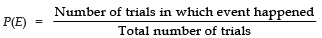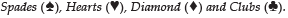Courses

# Facts that Matter- Probability Class 10 Notes | EduRev

## Class 10 : Facts that Matter- Probability Class 10 Notes | EduRev

The document Facts that Matter- Probability Class 10 Notes | EduRev is a part of the Class 10 Course Mathematics (Maths) Class 10.
All you need of Class 10 at this link: Class 10

Facts that Matter

• We know that experimental (or empirical) probabilities of events are based on the results of actual experiments.
• If we perform the same experiment for different number of times, we get different data giving different probability.
•where, P(E) is the experimental (or empirical probability).

Remember:
(i) The experimental or empirical probability of an event is based on what has actually happened whereas the theoretical probability of the event tries to predict what will happen on the basis of certain assumptions.
(ii) An experiment in which all possible outcomes are known in advance and the exact outcome of specific event cannot be predicted in advance, is called a random experiment. The word random means “all outcomes have equal chances of occurrence.“
(iii) Performing a random experiment is trial.
(iv) Die is a solid cube having six faces marked as 1, 2, 3, 4, 5, 6 respectively. Plural of die is dice.
(v) Events are said to be equally likely, if under the given condition, we cannot prefer one event to another event.
(vi) Favourable outcomes are those which result in occurrence of an event in question.
(vii) A pack of playing cards consists of four suits:Each suit has 13 cards (⇒ total cards 52).
Kings, Queens and Jacks are called Face-cards.
(⇒ There are 12-face cards in a pack)
(viii) If we toss a coin, two outcomes are possible : Head or Tail.
(ix) Probability of an impossible event = 0.
(x) Probability of a sure event = 1.
(xi) Probability of a possible event can be between 0 and 1.

Offer running on EduRev: Apply code STAYHOME200 to get INR 200 off on our premium plan EduRev Infinity!

,

,

,

,

,

,

,

,

,

,

,

,

,

,

,

,

,

,

,

,

,

;